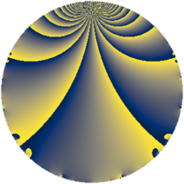# Properties

 Label 1690.2.aLevel $1690$ Weight $2$ Character orbit 1690.a Rep. character $\chi_{1690}(1,\cdot)$ Character field $\Q$ Dimension $53$ Newform subspaces $23$ Sturm bound $546$ Trace bound $7$

# Related objects

## Defining parameters

 Level: $$N$$ $$=$$ $$1690 = 2 \cdot 5 \cdot 13^{2}$$ Weight: $$k$$ $$=$$ $$2$$ Character orbit: $$[\chi]$$ $$=$$ 1690.a (trivial) Character field: $$\Q$$ Newform subspaces: $$23$$ Sturm bound: $$546$$ Trace bound: $$7$$ Distinguishing $$T_p$$: $$3$$, $$7$$, $$11$$, $$31$$

## Dimensions

The following table gives the dimensions of various subspaces of $$M_{2}(\Gamma_0(1690))$$.

Total New Old
Modular forms 300 53 247
Cusp forms 245 53 192
Eisenstein series 55 0 55

The following table gives the dimensions of the cuspidal new subspaces with specified eigenvalues for the Atkin-Lehner operators and the Fricke involution.

$$2$$$$5$$$$13$$FrickeDim
$$+$$$$+$$$$+$$$+$$$9$$
$$+$$$$+$$$$-$$$-$$$5$$
$$+$$$$-$$$$+$$$-$$$8$$
$$+$$$$-$$$$-$$$+$$$5$$
$$-$$$$+$$$$+$$$-$$$8$$
$$-$$$$+$$$$-$$$+$$$5$$
$$-$$$$-$$$$+$$$+$$$2$$
$$-$$$$-$$$$-$$$-$$$11$$
Plus space$$+$$$$21$$
Minus space$$-$$$$32$$

## Trace form

 $$53 q - q^{2} + 53 q^{4} - q^{5} - 4 q^{6} + 8 q^{7} - q^{8} + 57 q^{9} + O(q^{10})$$ $$53 q - q^{2} + 53 q^{4} - q^{5} - 4 q^{6} + 8 q^{7} - q^{8} + 57 q^{9} + q^{10} + 8 q^{11} + 4 q^{14} + 4 q^{15} + 53 q^{16} + 6 q^{17} + 3 q^{18} - q^{20} - 8 q^{23} - 4 q^{24} + 53 q^{25} + 8 q^{28} + 10 q^{29} + 8 q^{31} - q^{32} - 8 q^{33} - 10 q^{34} + 57 q^{36} - 6 q^{37} + 8 q^{38} + q^{40} - 14 q^{41} + 16 q^{42} + 8 q^{43} + 8 q^{44} + 3 q^{45} + 4 q^{46} + 16 q^{47} + 57 q^{49} - q^{50} - 8 q^{51} - 10 q^{53} + 8 q^{54} + 12 q^{55} + 4 q^{56} - 8 q^{57} - 6 q^{58} - 24 q^{59} + 4 q^{60} + 10 q^{61} + 16 q^{62} + 8 q^{63} + 53 q^{64} + 24 q^{66} + 12 q^{67} + 6 q^{68} + 16 q^{69} - 8 q^{70} + 8 q^{71} + 3 q^{72} - 10 q^{73} + 6 q^{74} - 24 q^{77} + 32 q^{79} - q^{80} + 77 q^{81} - 18 q^{82} - 12 q^{83} + 6 q^{85} + 12 q^{86} - 8 q^{87} + 10 q^{89} + 13 q^{90} - 8 q^{92} + 16 q^{93} + 4 q^{94} + 12 q^{95} - 4 q^{96} - 2 q^{97} + 7 q^{98} + 8 q^{99} + O(q^{100})$$

## Decomposition of $$S_{2}^{\mathrm{new}}(\Gamma_0(1690))$$ into newform subspaces

Label Dim $A$ Field CM Traces A-L signs $q$-expansion
$a_{2}$ $a_{3}$ $a_{5}$ $a_{7}$ 2 5 13
1690.2.a.a $1$ $13.495$ $$\Q$$ None $$-1$$ $$-2$$ $$-1$$ $$-1$$ $+$ $+$ $+$ $$q-q^{2}-2q^{3}+q^{4}-q^{5}+2q^{6}-q^{7}+\cdots$$
1690.2.a.b $1$ $13.495$ $$\Q$$ None $$-1$$ $$0$$ $$-1$$ $$0$$ $+$ $+$ $+$ $$q-q^{2}+q^{4}-q^{5}-q^{8}-3q^{9}+q^{10}+\cdots$$
1690.2.a.c $1$ $13.495$ $$\Q$$ None $$-1$$ $$0$$ $$-1$$ $$3$$ $+$ $+$ $+$ $$q-q^{2}+q^{4}-q^{5}+3q^{7}-q^{8}-3q^{9}+\cdots$$
1690.2.a.d $1$ $13.495$ $$\Q$$ None $$-1$$ $$2$$ $$-1$$ $$0$$ $+$ $+$ $-$ $$q-q^{2}+2q^{3}+q^{4}-q^{5}-2q^{6}-q^{8}+\cdots$$
1690.2.a.e $1$ $13.495$ $$\Q$$ None $$-1$$ $$2$$ $$1$$ $$4$$ $+$ $-$ $+$ $$q-q^{2}+2q^{3}+q^{4}+q^{5}-2q^{6}+4q^{7}+\cdots$$
1690.2.a.f $1$ $13.495$ $$\Q$$ None $$1$$ $$-2$$ $$-1$$ $$4$$ $-$ $+$ $+$ $$q+q^{2}-2q^{3}+q^{4}-q^{5}-2q^{6}+4q^{7}+\cdots$$
1690.2.a.g $1$ $13.495$ $$\Q$$ None $$1$$ $$-2$$ $$1$$ $$1$$ $-$ $-$ $+$ $$q+q^{2}-2q^{3}+q^{4}+q^{5}-2q^{6}+q^{7}+\cdots$$
1690.2.a.h $1$ $13.495$ $$\Q$$ None $$1$$ $$0$$ $$1$$ $$-3$$ $-$ $-$ $+$ $$q+q^{2}+q^{4}+q^{5}-3q^{7}+q^{8}-3q^{9}+\cdots$$
1690.2.a.i $1$ $13.495$ $$\Q$$ None $$1$$ $$2$$ $$1$$ $$0$$ $-$ $-$ $-$ $$q+q^{2}+2q^{3}+q^{4}+q^{5}+2q^{6}+q^{8}+\cdots$$
1690.2.a.j $2$ $13.495$ $$\Q(\sqrt{3})$$ None $$-2$$ $$-2$$ $$2$$ $$6$$ $+$ $-$ $-$ $$q-q^{2}+(-1+\beta )q^{3}+q^{4}+q^{5}+(1+\cdots)q^{6}+\cdots$$
1690.2.a.k $2$ $13.495$ $$\Q(\sqrt{10})$$ None $$-2$$ $$0$$ $$2$$ $$2$$ $+$ $-$ $+$ $$q-q^{2}+\beta q^{3}+q^{4}+q^{5}-\beta q^{6}+q^{7}+\cdots$$
1690.2.a.l $2$ $13.495$ $$\Q(\sqrt{3})$$ None $$-2$$ $$2$$ $$2$$ $$-2$$ $+$ $-$ $+$ $$q-q^{2}+(1+\beta )q^{3}+q^{4}+q^{5}+(-1+\cdots)q^{6}+\cdots$$
1690.2.a.m $2$ $13.495$ $$\Q(\sqrt{3})$$ None $$2$$ $$-2$$ $$-2$$ $$-6$$ $-$ $+$ $-$ $$q+q^{2}+(-1+\beta )q^{3}+q^{4}-q^{5}+(-1+\cdots)q^{6}+\cdots$$
1690.2.a.n $2$ $13.495$ $$\Q(\sqrt{10})$$ None $$2$$ $$0$$ $$-2$$ $$-2$$ $-$ $+$ $+$ $$q+q^{2}+\beta q^{3}+q^{4}-q^{5}+\beta q^{6}-q^{7}+\cdots$$
1690.2.a.o $2$ $13.495$ $$\Q(\sqrt{3})$$ None $$2$$ $$2$$ $$-2$$ $$2$$ $-$ $+$ $+$ $$q+q^{2}+(1+\beta )q^{3}+q^{4}-q^{5}+(1+\beta )q^{6}+\cdots$$
1690.2.a.p $3$ $13.495$ $$\Q(\zeta_{14})^+$$ None $$-3$$ $$-1$$ $$3$$ $$-2$$ $+$ $-$ $+$ $$q-q^{2}-\beta _{1}q^{3}+q^{4}+q^{5}+\beta _{1}q^{6}+\cdots$$
1690.2.a.q $3$ $13.495$ $$\Q(\zeta_{14})^+$$ None $$-3$$ $$1$$ $$3$$ $$-5$$ $+$ $-$ $-$ $$q-q^{2}+\beta _{1}q^{3}+q^{4}+q^{5}-\beta _{1}q^{6}+\cdots$$
1690.2.a.r $3$ $13.495$ $$\Q(\zeta_{14})^+$$ None $$3$$ $$-1$$ $$-3$$ $$2$$ $-$ $+$ $-$ $$q+q^{2}-\beta _{1}q^{3}+q^{4}-q^{5}-\beta _{1}q^{6}+\cdots$$
1690.2.a.s $3$ $13.495$ $$\Q(\zeta_{14})^+$$ None $$3$$ $$1$$ $$-3$$ $$5$$ $-$ $+$ $+$ $$q+q^{2}+\beta _{1}q^{3}+q^{4}-q^{5}+\beta _{1}q^{6}+\cdots$$
1690.2.a.t $4$ $13.495$ 4.4.4752.1 None $$-4$$ $$2$$ $$-4$$ $$0$$ $+$ $+$ $-$ $$q-q^{2}+(1+\beta _{2}-\beta _{3})q^{3}+q^{4}-q^{5}+\cdots$$
1690.2.a.u $4$ $13.495$ 4.4.4752.1 None $$4$$ $$2$$ $$4$$ $$0$$ $-$ $-$ $-$ $$q+q^{2}+(1+\beta _{2}-\beta _{3})q^{3}+q^{4}+q^{5}+\cdots$$
1690.2.a.v $6$ $13.495$ 6.6.20439713.1 None $$-6$$ $$-2$$ $$-6$$ $$-3$$ $+$ $+$ $+$ $$q-q^{2}-\beta _{1}q^{3}+q^{4}-q^{5}+\beta _{1}q^{6}+\cdots$$
1690.2.a.w $6$ $13.495$ 6.6.20439713.1 None $$6$$ $$-2$$ $$6$$ $$3$$ $-$ $-$ $-$ $$q+q^{2}-\beta _{1}q^{3}+q^{4}+q^{5}-\beta _{1}q^{6}+\cdots$$

## Decomposition of $$S_{2}^{\mathrm{old}}(\Gamma_0(1690))$$ into lower level spaces

$$S_{2}^{\mathrm{old}}(\Gamma_0(1690)) \cong$$ $$S_{2}^{\mathrm{new}}(\Gamma_0(169))$$$$^{\oplus 4}$$$$\oplus$$$$S_{2}^{\mathrm{new}}(\Gamma_0(26))$$$$^{\oplus 4}$$$$\oplus$$$$S_{2}^{\mathrm{new}}(\Gamma_0(65))$$$$^{\oplus 4}$$$$\oplus$$$$S_{2}^{\mathrm{new}}(\Gamma_0(130))$$$$^{\oplus 2}$$$$\oplus$$$$S_{2}^{\mathrm{new}}(\Gamma_0(338))$$$$^{\oplus 2}$$$$\oplus$$$$S_{2}^{\mathrm{new}}(\Gamma_0(845))$$$$^{\oplus 2}$$